# Application of a weighted likelihood method to hypocenter determination

## Abstract

The method of least squares is a standard approach to hypocenter determination in seismology. However, this method is not useful for data contaminated by systematic errors. To address this problem, we propose a weighted likelihood method (WLL) rather than a weighted least-squares method (WLSQ). Assuming a normally distributed random error and systematic errors, both methods give the same solution; however, variances of random errors estimated by WLSQ are much smaller than those estimated by WLL. Examining reasonable random errors, we simulate a case of systematic errors varying linearly with a given parameter, where the number of unknown parameters is reduced to one for simplification. We assume that a systematic error, two different arrays of stations, and three different weights are functions of distance. In the cases where biases affected by systematic errors are adequately reduced, the variances of random errors estimated by WLL become roughly equal to that assumed, but those estimated by WLSQ are much smaller than that assumed. This result implies that WLL is a better approach than WLSQ for data contaminated by systematic errors.

## 1. Introduction

The method of least squares is a standard approach to the approximate solution of overdetermined systems. Use of this method can reduce the effect of random errors. The best solution in the least-squares method minimizes the sum of squared residuals—a residual being the difference between an observed value (arrival time of a seismic wave) and the fitted value provided by a model (origin time and location of an earthquake and a seismic velocity model). Hypocenter determination employs the nonlinear least squares method and is implemented by iterative refinement. If it is assumed that random error variances largely vary among observation stations, the weighted least-squares method (WLSQ) can be used to determine the hypocenter more reliably.

The residual is caused by both errors in the measurement of the arrival time and errors in the seismic velocity model for calculating theoretical arrival times. The former is a random error, but the latter is a systematic one. For a nationwide network in Japan, such as those operated by the Japan Meteorological Agency (JMA) and the National Research Institute for Earth Science and Disaster Prevention (NIED), a simple velocity model for hypocenter determination often involves systematic errors in the calculated travel time, since seismic velocities vary from region to region. JMA and NIED currently adopt WLSQ, in which the weight of each observation depends on its hypocentral distance. Their procedure, which may be a better approach than a simple least-squares method, obviously violates the condition that the least-squares method must be applied to data without systematic errors. Therefore, this procedure may give unreliable solutions, which has not yet been addressed.

In this study, we presume both normally distributed random errors and systematic errors and propose the use of the weighted likelihood method (WLL) (Hu and Zidek, 2002; Wang and Zidek, 2005) to address this problem. First, we demonstrate that WLSQ and WLL provide the same solution; however, the variance of random errors estimated by WLL exceeds that estimated by WLSQ. In order to clarify which method estimates more reasonable errors, both methods are applied to data contaminated with systematic errors in order to simulate hypocenter determination with an unsuitable velocity model. The solutions with both methods could be analytically obtained for the simulated data. This paper compares the variance of random errors estimated by the maximum likelihood method with the assumed ones. This comparison indicates that WLSQ underestimates the variance of the random errors and is unreliable as an approach to this problem.

## 2. Method

### 2.1 Weighted least squares

The simple least-squares method assumes that the standard deviation of the random error is constant for all samples. When the standard deviation varies, WLSQ can be applied. Less weight is given to less precise measurements, and more weight is given to more precise measurements. Here, σ and σ i denote the standard deviation of the random error in a representative observation and the ith observation (i = 1,… n), respectively. The weight w i is inversely proportional to the variance of the error, and is represented by. The likelihood function in this case is given as:((1))

where o i denotes the observed value and c i denotes the theoretical value at the i-th observation.

The maximum log-likelihood is obtained as:((2))

where((3))

whereis the minimum of R2.

The maximum likelihood estimate of,, is given by:((4))

Then((5))

where the subscript WLSQ refers to the weighted least-squares method.

### 2.2 Weighted likelihood method

If reliabilities of observed samples differ from sample to sample, each sample can contribute to the maximum likelihood estimate in proportion to its reliability through WLL. The WLL function is given in the form of the weighted sum of log-likelihoods for each sample: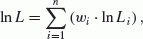((6))((7))

The maximum log-likelihood is obtained as:((8))

The maximum likelihood estimate of σ2,, is given by((9))

Then((10))

where the subscript WLL refers to the weighted likelihood method.

When the same weights are applied in WLL and in WLSQ, the same solutions of c i are obtained. However, the maximum likelihood estimates of σ2 are different.

## 3. Simulation

In applying WLL to hypocenter determination, the only difference from WLSQ is related to the formula for σ2, where Eq. (10) can be compared with Eq. (5). When NIED and JMA determine the hypocenter, a weight with epicentral distance may be introduced to reduce bias due to systematic errors in the calculated travel times. The structure of seismic wave velocities in Japan cannot be modeled with a simple layered structure, as employed by NIED and JMA. Therefore, using arrival times of more distant stations in the determination results in systematic errors, and bias of the determined hypocenter. In this section, we examine which of WLSQ or WLL is better for hypocenter determination affected by systematic errors from an inappropriate velocity structure.

We schematically simulate this problem assuming simple conditions. We assume that an earthquake occurs on the surface of a uniform medium and that stations are densely deployed in a line from the epicenter in the first case, and on a two-dimensional surface in the second case. A systematic error due to an inappropriate velocity structure is as large as a random error at a short distance, and becomes much larger at more distant points. It is assumed that the epicenter of the earthquake is rather well constrained but the origin time of the earthquake is not, since the dense stations are well distributed. Thus, the problem of hypocenter determination is reduced to the problem of determining the origin time of the event.

The observed arrival time at the ith (i = 1, 2, ..n) station is((11))

where r i is the epicentral distance, T(r i ) is the travel time estimated by the model, and B(r i ) is a systematic error due to an inappropriate velocity model. In the single-layer model, this correction must be a function of epicentral distance, seismic wave velocity in the model, and the actual seismic wave velocity:((12))

where V c is the actual value of the seismic wave velocity, and V m is the seismic wave velocity used in the model. The term on the right-hand side is a linear function of epicentral distance: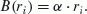((13))

Equation (11) can be represented as:((14))

As the epicenter is well constrained under our assumption, the epicentral distance is considered to be a known parameter. Therefore, subtracting T (r i ) from the arrival time, we consider t0 to be an unknown parameter, and Eq. (14) can be replaced by:((15))

Here, the problem of hypocenter determination is reduced to the problem of estimating t0 from observation o i contaminated by a systematic error and a random error.

Without loss of generality, we fix the true value of the origin time to be 0. The sum of the residual squares R2 is calculated as:((16))

where x refers to the estimated origin time. The maximum likelihood estimate of the origin time,, minimizes R2 and is calculated as follows:((17))

The minimum of R2 is calculated as:((18))

In the present study, we can estimate the expected values of Eqs. (17) and (18) with a probability density function for the epicentral distance of stations, S(r), and the assumption that the random error of ε i is normally distributed with a mean 0 and variance δ2. A weight function W(r) is applied and different limits of epicentral distance, u, are considered.

In order to derive an analytical solution of Eq. (17), we assume a two-step random value generation.

Step 1. Random generation of n stations within a distance of u.

Step 2. For each set of stations, generate a large number (l) of series of random errors, (ε ij : where j refers to the number of trials, j = 1, 2,…l).

For the jth trial of ε ij , we obtain the maximum likelihood estimate of the origin timeas follows:((19))

The average value of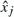over j,, is estimated by:((20))

where ε ij refers to the random error at the ith station in the jth trial. For a large number of l,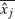approachesas:((21))

where E denotes the expected value of a variable, since, for large enough values of l,((22))

which is an average of independent random errors ε ij normally distributed with a mean 0. Thus, for each selection of stations, the expected value of Eq. (17) is given by Eq. (21).

For a set of large n, Eq. (21) can be represented as:

$$E(\hat x) = \frac{A}{C},$$
((23))

where:((24))((25))((26))

and((27))

Here, we assume that m stations are distributed within an epicentral distance of 10 units.

Similarly, the expected value of Eq. (18) is derived as follows. For the jth trial of a set of stations: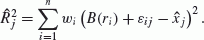((28))

For a set of large n,is replaced withof Eq. (21). This replacement will be justified by a Monte Carlo simulation. We obtain the average value of Eq. (28) over j for a large number of l:((29))

Equation (29) can be represented by:((30))

where((31))

We analytically estimated the expected values ofandfor a large number of n in this way. Hereafter,anddenote the expected values ofand, respectively.

We consider two different observation station arrays and three different weight functions. In one case of the arrays, these stations are distributed in a line (Array1). The density function of S(r) is given as:((32))

In the other case, we consider stations deployed uniformly in a plane (Array2). The density function of S(r) is given as((33))

Three different weighting functions are considered below. For the first case of applying a constant weight, the function of W(r) is given as:((34))

For the second case, where the weight decays inversely as the second power of distance, the weight is given as:**((35))

The assumed systematic error, B(r), increases linearly with epicentral distance. WLSQ likely misapplies a weighting function that decays as the inverse of the second order of the epicentral distance, since the square of the systematic error increases in proportion to the second power of distance.

The last case, where the weight function decays as the inverse of the fourth power of epicentral distance, can be considered as an example of weight decaying faster than in the previous cases:((36))

Table 1 lists formulas estimating the values of A, C, and D for each of the six cases. Substituting these values into Eqs. (23), (30), and (5) or (10), we obtain standard deviations of random errors,for WLSQ andfor WLL.

Figures 1(a) through (f) illustrateandwith the upper limit u varying from 1 to 10 units, where α = 1 and δ = 1. The systematic error is assumed to be equal to, or less than, the random error in a range of 0 to 1 units, where the weight is fixed to 1.(dotted line) and(dashed line) are compared with the assumed random error (δ = 1). In each set,represents the bias affected by the systematic error, since the correct origin time is set to 0.

In Figs. 1(a), 1(b), 1(d) and 1(e), biases caused by systematic errors are not adequately reduced, wheredeparts from 0. In these cases,exceeds the standard deviation of the assumed random error. In both station arrays, it is clear that the most quickly decaying weight function (Figs. 1(c) and 1(f)), gives the best solutions, wherebecomes at most 1 even in cases including stations up to 10 units away. This suggests that biases are adequately reduced. In these cases,becomes reasonable at around 1, mostly equal to the standard deviation of the assumed random error. In contrast,values are much smaller than that assumed. Therefore, WLSQ underestimates the standard deviation of random errors, suggesting an invalid application of WLSQ to the present issue.

Table 2 summarizesandfor u = 10. Results obtained from the weight function decaying as the inverse of the seventh power are added as a more quickly decaying weight function, which is the case of NIED. In this case,andbecome slightly better than those of other cases, wherebecomes smaller andapproaches 1. These examples suggest that ifexceeds the standard deviation of the assumed random error, biases caused by systematic errors would not be adequately reduced.

Biascould not be compared with its standard error, the derivation of which is based on the Hessian/Fisher Information matrix of the log-likelihood function (Sakamoto et al., 1983), since the standard error ofconcerns only random errors, while the bias originates from systematic errors. From a quantitative viewpoint, it should be noted that, in our definition,andare functions of u and are independent of m, but the standard error ofdivided by the square root of C, is inversely proportional to the square root of m, which can easily be verified from Eqs. (5), (10), (23), (24), (25), (26), (30) and (31). The ratio ofto its standard error becomes large in proportion to the square root of m. Consequently, bias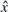cannot be compared with its standard error.Table 2. List of and . The listed values are estimated by stations at distances of up to 10 units. Results from the 7th power are also listed.

## 4. Discussion and Summary

A Monte Carlo simulation was conducted to confirm Eq. (23) and justify the replacement in Eq. (29). Figures 2(a), 2(b) and 2(c) illustrateandvalues obtained for the set of Fig. 1(f). For each distance limit, the number of stations can be calculated by S(r), and their locations are randomly generated. Random errors are also generated once for each station, which implies l = 1 in Eqs. (20) and (29). Ten thousand sets of stations are generated for each distance limit, which is shifted from 1 to 10 units for every unit step. In each set of the figure, the simple averages over ten thousand sets for m = 200 (dashed line) and m = 500 (dotted line) are compared with analytical solutions (solid line).

In general, as the number of stations increases (fixed distance limit), the results obtained by the simulation approach those of the analytical solution. Qualitative relations amongandobtained from the Monte Carlo simulation do not largely differ from those of the analytical solutions, except in the case of a few tens of stations. Although not shown here, the agreement between the simulation and the analytical solution in other cases (the other station array and/or different weights) becomes better than that of Fig. 2. We have only discussed qualitative relations between the parameters, so an analytical solution is an appropriate approach to this issue.

Ueno et al. (2002) reported that hypocenters of larger earthquakes tend to be deeper than those of smaller ones. They speculated that this tendency is related to the ratio of the number of long-distance stations to that of short-distance stations. This implies that a bias of hypocenters can be caused by systematic errors depending on the epicentral/hypocentral distance, which originate from the difference in velocity structure between the model used in hypocenter determination and the actual case. In order to reduce this bias, NIED (Shiomi, 2009 (personal communication)) introduced a weight depending on the hypocentral distance, Y:where Ymin is the hypocentral distance of the station closest to the hypocenter (km) (if Ymin ≤ 50, then Ymin = 50; if W(Y) > 1, then W(Y) = 1).

However, JMA introduced a similar weighting depending on the hypocentral distance except for the order of power:

For P-waves (W p ):For S-waves (W s ): W s = W p /3.

Comparing weights in these methods with those of our simulation indicates that NIED, using a weight function decaying faster than the inverse fourth power, probably succeeds in reducing the bias by systematic errors (Table 2). But, JMA may fail to reduce bias with a weight function decaying inversely with the second power of distance. Both NIED and JMA must underestimate standard errors of hypocenters, which are calculated based on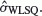. Standard errors of hypocenters are often critical in considering reliable hypocenter distributions of clustering earthquakes and their tectonic implications.

For deeper earthquakes, a qualitative consideration could be given as follows. At short epicentral distances compared with the focal depth, systematic errors reach a level depending on the focal depth. On the other hand, at longer distances, systematic errors increase with distance mostly similar to those of shallow earthquakes. These result in smaller variances of residuals than those of shallower earthquakes, since a range of systematic errors becomes smaller than those of shallower earthquakes. This implies that a significant bias may remain, even ifapproaches the variance of random error. Such aspects should be discussed further as separate studies, since station arrays, the number of unknown parameters, and other factors must be rearranged to address these issues.

The present paper focuses on only the preliminary formulation of applying WLL to hypocenter determinations. In practical applications of WLL, an optimal weight function should be determined taking into consideration the results in the case of various kinds of earthquakes in different areas and depths (Hu and Zidek, 2002). WLL can be applied to hypocenter determination with a minor revision of current methods which are based on misapplications of WLSQ.

We propose the use of WLL rather than WLSQ for hypocenter determination. Both methods give the same solution; however, the variance estimated by WLSQ is much smaller than that estimated by WLL. Our simulation indicates that a weight function that decays faster with distance gives a better solution, which could be realized by WLL. In contrast, such flexible weight functions are not justified by WLSQ, since weights should be inversely proportional to the variances of errors. In the cases where biases affected by systematic errors are adequately reduced, the variances of random errors estimated by WLL become roughly equal to the given one, but those estimated by WLSQ are much smaller than the given one. Therefore, WLSQ should not be used to address systematic errors in hypocenter determination. We conclude that WLL is a better approach than WLSQ for data contaminated by systematic errors.

## References

• Hu, F. and J. V. Zidek, The weighted likelihood, Can. J. Stat., 30, 347–371, 2002.

• Japan Meteorological Agency, Users’ Guide, http://data.sokki.jmbsc.or.jp/cdrom/seismological/catalog/notese.htm (as of April 1, 2013).

• Sakamoto, Y., M. Ishiguro, and G. Kitagawa, Akaike Information Criterion Statistics, 290 pp, D. Reidel, Dordrecht, 1983.

• Ueno, H., S. Hatakeyama, J. Funakaki, and N. Hamada, Improvement of hypocenter determination procedures in the Japan Meteorological Agency, Quart. J. Seismol., 65, 123–131, 2002 (in Japanese).

• Wang, X. and J. V. Zidek, Derivation of mixture distributions and weighted likelihood function as minimizers of KL-divergence subject to constraints, Ann. Inst. Statist. Math., 57, 687–701, 2005.

## Acknowledgments

The author thanks two anonymous reviewers for their critical reading and comments on this manuscript.

## Author information

Authors

### Corresponding author

Correspondence to M. Imoto.

## Rights and permissions

The images or other third party material in this article are included in the article’s Creative Commons licence, unless indicated otherwise in a credit line to the material. If material is not included in the article’s Creative Commons licence and your intended use is not permitted by statutory regulation or exceeds the permitted use, you will need to obtain permission directly from the copyright holder.

To view a copy of this licence, visit https://creativecommons.org/licenses/by/4.0/.

Reprints and Permissions

Imoto, M. Application of a weighted likelihood method to hypocenter determination. Earth Planet Sp 65, 1569–1574 (2013). https://doi.org/10.5047/eps.2013.07.010

• Revised:

• Accepted:

• Published:

• Issue Date:

• DOI: https://doi.org/10.5047/eps.2013.07.010

### Key words

• Weighted likelihood method
• weighted least squares
• hypocenter determination
• systematic error